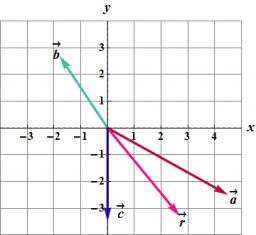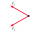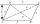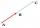# Three vectors

The three forces whose amplitudes are in ratio 9:10:17 act in the plane at one point to balance. Determine the angles of each two forces.

A =  53.1301 °
B =  154.9424 °
C =  151.9275 °

### Step-by-step explanation:

$B=180-{u}_{1}=180-25.0576=154.9424\text{°}=154\mathrm{°}5{6}^{\mathrm{\prime }}33\mathrm{"}$
$C=180-{u}_{2}=180-28.0725=151.9275\text{°}=151\mathrm{°}5{5}^{\mathrm{\prime }}39\mathrm{"}$

Try calculation via our triangle calculator.Did you find an error or inaccuracy? Feel free to write us. Thank you!Tips to related online calculators
Line slope calculator is helpful for basic calculations in analytic geometry. The coordinates of two points in the plane calculate slope, normal and parametric line equation(s), slope, directional angle, direction vector, the length of the segment, intersections of the coordinate axes, etc.
Check out our ratio calculator.
Our vector sum calculator can add two vectors given by their magnitudes and by included angle.
Cosine rule uses trigonometric SAS triangle calculator.

#### You need to know the following knowledge to solve this word math problem:

We encourage you to watch this tutorial video on this math problem:

## Related math problems and questions:

• ForcesForces with magnitudes F1 = 42N and F2 = 35N act at a common point and make an angle of 77°12'. How big is their resultant?
• Vector sumThe magnitude of the vector u is 12 and the magnitude of the vector v is 8. Angle between vectors is 61°. What is the magnitude of the vector u + v?
• Space vectors 3DThe vectors u = (1; 3; -4), v = (0; 1; 1) are given. Find the size of these vectors, calculate the angle of the vectors, the distance between the vectors.
• Two forcesTwo forces with magnitudes of 25 and 30 pounds act on an object at 10° and 100° angles. Find the direction and magnitude of the resultant force. Round to two decimal places in all intermediate steps and your final answer.
• Two forcesThe two forces F1 = 580N and F2 = 630N, have an angle of 59 degrees. Calculate their resultant force, F.
• Mass pointTwo equal forces of 30 Newtons act on a mass point. Find the magnitude of the resultant force if these forces form an angle of 42°.
• TrianglePlane coordinates of vertices: K[11, -10] L[10, 12] M[1, 3] give Triangle KLM. Calculate its area and its interior angles.
• CuboidsTwo separate cuboids with different orientation in space. Determine the angle between them, knowing the direction cosine matrix for each separate cuboid. u1=(0.62955056, 0.094432584, 0.77119944) u2=(0.14484653, 0.9208101, 0.36211633)
• Angles by cosine lawCalculate the size of the angles of the triangle ABC, if it is given by: a = 3 cm; b = 5 cm; c = 7 cm (use the sine and cosine theorem).
• Resultant forceCalculate mathematically and graphically the resultant of three forces with a common center if: F1 = 50 kN α1 = 30° F2 = 40 kN α2 = 45° F3 = 40 kN α3 = 25°
• Angle between vectorsFind the angle between the given vectors to the nearest tenth of a degree. u = (-22, 11) and v = (16, 20)
• Inner anglesThe inner angles of the triangle are 30°, 45° and 105° and its longest side is 10 cm. Calculate the length of the shortest side, write the result in cm up to two decimal places.
• ForcesIn point O acts three orthogonal forces: F1 = 20 N, F2 = 7 N, and F3 = 19 N. Determine the resultant of F and the angles between F and forces F1, F2, and F3.
• BearingA plane flew 50 km on a bearing 63°20' and the flew on a bearing 153°20' for 140km. Find the distance between the starting point and the ending point.
• ParallelogramThe sides of the parallelogram are 8 cm and 6 cm long and the angle of the diagonals is 60°. What is its area?
• VectorsVector a has coordinates (8; 10) and vector b has coordinates (0; 17). If the vector c = b - a, what is the magnitude of the vector c?
• Linear independenceDetermine if vectors u=(-4; -10) and v=(-2; -7) are linear dependent.